Appearance

# TypeScript 中你可能会忽略的细节

WARNING

## # 回顾下 TS 简单用法

``````let a: string = 'hello ts' // 显示注解声明
let b = 'hello ts' // ts自动推导
type A = string // 类型别名
interface Person { // 接口
name: string
age: number
count: number
friends: {
name: string
age: number
}[]
}
interface Shinji extends Person {
Auto: string
}
enum Weekday { // 枚举
Mon,
Tue,
Wed,
// ....
}
let tuple:[number, string] = [1, '2'] // 元组

interface Nerv {
attack(target: string): void
}
class EVA implements Nerv { // 类与接口实现implements
private readonly RobotType: string = 'Zero'
attact(target: string) {
console.log(target)
}
}
``````

## # 那些你可能忽视的细节

### # const 声明的类型推导

ts 作为一种使用了两种类型系统实现的语言，既可以通过注解显示声明类型，也可以通过 ts 自动推导类型。请看下面例子，参数`a`的值通过 ts 类型推导，推导为 `boolean` 类型， 那么参数`b` ts 是否会自动推导出来是 `boolean` 类型呢？

``````let a = true // boolean
const b = true // => ?
``````

``````const a = {
b: 12
}
``````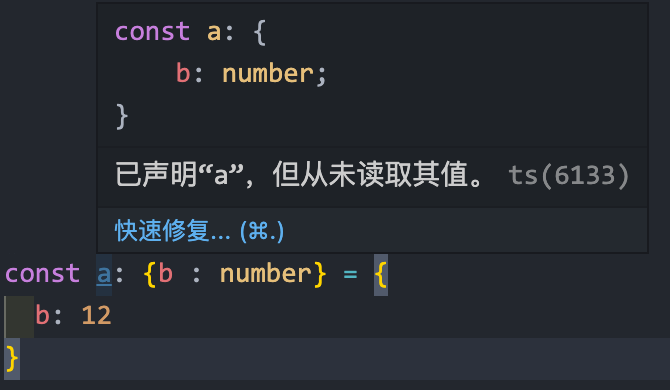这里很奇怪的是 ts 推导出来的 `b` 居然是一个 `number` 类型，而不是字面量 `12`。上面第一个例子来说 `const` 或者 `let` 声明 是对 ts 推导有影响的，这些都是作用于基本类型，使用 `const` 声明对象的话，ts 的推导就不会缩窄，这条规则也就失效了。这种情况类似 esnext 中的 `const` 声明。

### # 元组

``````let a: [number] = 
let b : [number, string] = [1, '2']
``````

``````let c: [number, string?] = 
``````

``````let d: [string, ...string[]] = ['shinji', 'rei', 'asuka']
let e: [number, boolean, ...string[]] = [3, true, 'hello', 'ts']
``````

``````let a = [1, true] // 没有显示注解声明，猜一下ts会自动推导出来什么？
``````

• 方式一：
``````let a = [1, true] as [number, boolean]
``````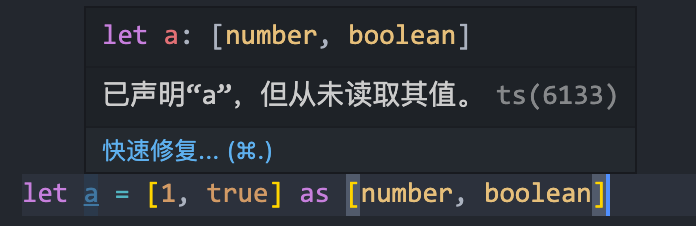• 方式二： 这种 `as const` 的方式有副作用，会把类型置为 `readonly` 并且类型变为 `const` 类型， 即 `a` 的实际类型为： `readonly [1, true]`
``````let a = [1, true] as const
``````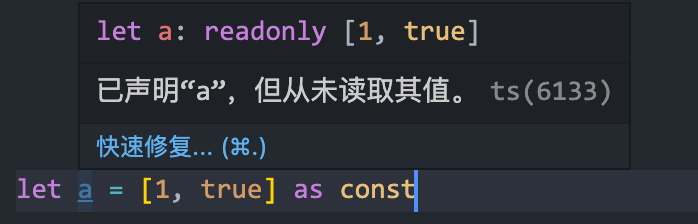• 方式三： 我们可以使用 ts 推导剩余参数的类型方式，将元组的类型收窄
``````function tuple<T extends unknown[]>(...args: T): T {
return args
}
let a = tuple(1, true)
``````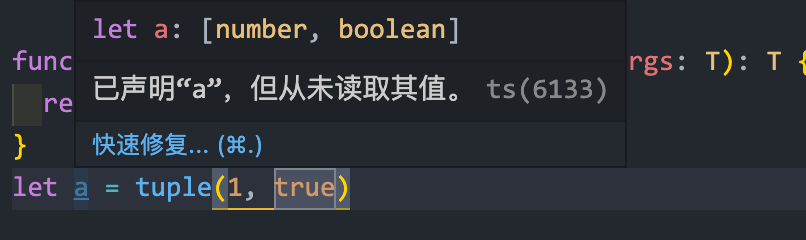这里的关键在于 `...args: T` ，由于范型 T描述的是剩余参数，因此 ts 推导出来是一个元组类型。 当项目中使用了大量的元组类型，但又不想使用 ts 默认的类型推导，我们就可以利用这个技术。

### # 枚举

1 个枚举可以分成多次声明，ts 会自动将其合并，和 `interface` 自动合并一个效果。

``````enum Language {
Chinese,
English,
Spanish
}
enum Language {
Russian = 3
}
// 两处枚举会自动合并
``````

ts 较为灵活，允许通过值访问枚举，也可以通过键访问枚举，但是容易出现两种问题

``````let langA = Language.Chinese
let langB = Language.Japanese // Error

let langC = Language
let langD = Language // 实际上Language不存在，但是ts居然不报错
``````

``````const enum Language {
Chinese,
English,
Spanish,
Russian
}

let langA = Language.Chinese
let langB = Language.Japanese // Error ts 会提示报错

let langC = Language // Error ts 会提示报错
let langD = Language // Error ts 会提示报错
// 以上具体报错类型，请自行在IDE中尝试
``````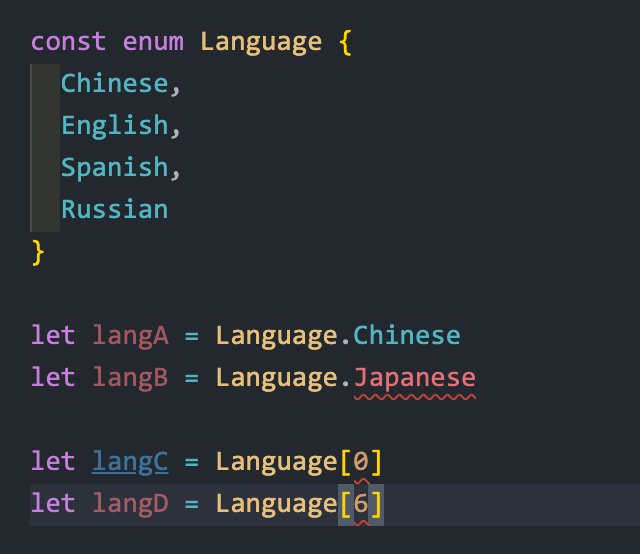使用安全子集的话该报错的就都会提示了，原因是：`const enum` 不允许反向查找，此行为与普通的 JavaScript 对象很像。而且使用了 `const enum` 后，ts 是不会生成 js 代码的，此功能谨慎使用。如果想在使用 `const enum` 的同时又生成 js 代码，请在 `ts.config.js` 中开启 `preserveConstEnums` 选项。

### # TS 中 this 类型注解

`this` 在 js 中是一个很特殊的存在，这里不过多的讨论 `this` 的用法，来聊一下 `this` 除了可以作为值 之外，还可以作为类型。

``````// this 不是常规的参数，是保留字，是函数签名的一部分
function fancyDate(this: Date){
return `\${this.getDate()}/\${this.getMonth()}/\${this.getFullYear()}`
}
// 如果这么调用，ts 将会提示报错
fancyDate()
// 正确的调用方式
fancyDate.call(new Date())
``````对于类来说，`this` 的类型也可以用于注解的方法返回类型。

``````let set = new Set()
set.has(2) // true
set.has(3) // false
``````

``````class SimpleSet {
// has方法
has(value: number): boolean {
// TODO:
}
// 返回的一定是一个 Set 类型的数据结构
// TODO:
}
}
``````

``````class SonOfSimpleSet extends SimpleSet{
has(value: number): boolean {}
}
``````

``````class SimpleSet {
has(value: number): boolen {}
}
// 这样的话，我们就可以不用在 SonOfSimpleSet 类中重复定义 add 方法了。在 SimpleSet
// 中 this 就会指向 SimpleSet，在 SonOfSimpleSet 中 this 就会指向 SonOfSimpleSet
``````

### # 范型 `T`

``````type Filter = {
<T>(array: T[], f: (item: T) => void): T[]
}
let filter: Filter = (array, f) => //....
// 范型 T 被绑定为 number
filter([1, 2, 3], _ => _ > 2)
// 范型 T 被绑定为 string
filter(['a', 'b'], _ => _ !== 'b')
``````

``````// 这个例子中，范型 T 被声明在具体的函数调用签名中，那么 ts 将会在 调用 Filter 类型的函数
// 时为范型 T 绑定具体的类型
type Filter = {
<T>(array: T[], f: (item: T) => void): T[]
}
let filter: Filter = (array, f) => //....

// 如果范型 T的作用域限定在了类型别名 Filter 中，那么 ts 就会要求在使用 Filter 时显示
// 绑定类型
type Filter<T> = {
(array: T[], f: (item: T) => boolean) : T[]
}
let filter: Filter = (array, f) => //.... Error TS2314: Generic type 'Filter'
// requires 1 type argument(s)
let filter: Filter<number> = (array, f) => // .... Good!
``````

``````type Filter = {
<T>(array: T[], f: (item: T) => void): T[]
}

type Filter<T> = {
(array: T[], f: (item: T) => boolean) : T[]
}

type Filter = <T>(array: T[], f(item: T) => boolan): T[]

type Filter<T> = (array: T[], f(item: T) => boolan): T[]

function filter<T>(array: T[], f(item: T) => boolean): []

class Filter<T, U> {}
// ....
``````

### # 快速区分 `void`、`any`、`unknown`、`never`

``````// void 此函数是一个返回 void 的函数
function returnVoid() {
let a = 1 + 1
let b = 2 + 2
}
// undefined 此函数是一个返回 undefined 的函数
function returnUndefined() {
return undefined
}
// null 此函数返回的是一个 数字或者 null
function returnNumOrNull(x: number) {
if (number < 10) return x
return null
}
// never 此函数返回的是 never
function returnNever() {
throw TypeError('always error')
}
// 另一个返回 never 的函数，无限循环
function returnNever2 () {
while(true) {
console.log('return never')
}
}
``````

### # 快速了解型变

``````type MyExclusive<T, U> = (T extends U ? never : T) | (U extends T ? never : U)
``````

``````// 1. 协变
T <: U
// 2. 不变
T 只能是 T
// 3. 逆变
T >: U
// 4. 双变
T <: U || T >: U // 即两种之一
``````

### # 函数类型重载

``````// 简写
type Log = (msg: string, userId?: string): void
// 完整型
type Log = {
(msg: string, userId?: string): void
}
``````

``````type Reserve = {
(from: Date, to: Date, destination: string): void
(from: Date, destination: string): void
}
let reseve: Reserve = (from, to, destination) => {}
``````

``````let reserve: Reserve =
(from: Date, toOrDestination: Date | string, destination?: string) => {}
``````

### # 伴生对象模式

``````
class Eva {}
let eva: Eva = new Eva() // 这一行代码中 第一个 Eva 指的是Eva类的实例类型，第二个Eva指
// 的是 Eva 这个值。

enum Nerv {
Zero,
One
}
let nerv: Nerv = Nerv.Zero // 这里第一个Nerv 指的是 Nerv枚举的类型， 第二 Nerv 指的
// 是 Nerv 这个值
``````

``````// a.ts
type Currency = {
unit: 'USD' | 'EUR' | 'JPY' | 'CNY',
value: number
}

let Currency = {
DEFAULT: 'USD',
from: (value: number, unit: Currency['unit']='USD'):Currency =>{
return {
unit,
value
}
}
}
export { Currency }
// b.ts
import { Currency } from './a.ts'
let amount: Currency = {
unit: 'CNY',
value: 79729
}
const c: Currency = Currency.from(100, 'EUR')
``````

• 可以语义上归属统一名称的类型和值
• 使用方可以一次性导入两者 除了可以将伴生对象模式用到值和类型别名外，接口和命名空间也可以使用伴生对象模式。

### # 安全的扩展原型

1. 比如在 zip.ts 文件中扩展 Array 的原型
2. 新增 zip 方法，增强原型功能。 代码：
``````// zip.ts
interface Array<T> {
zip<U>(list: U[]): [T, U][]
}

Array.prototype.zip = function<U, T>(
this: T[],
list: U[]
): [T, U][] {
return this.map((v, k)=> {
tuple(v, list[k])
})
}

function tuple<T extends unknown[]>(...args: T): T {
return args
}
``````

### # 非空断言

``````type Dialog = {
id?: string
}
// 使用非空断言
function cloneDialog(dialog: Dialog) {
if (!dialog.id) {
return
}
setTimeout(()=> {
removeFromDOM(
dialog,
document.getElementById(dialog.id)
)
}, 10)
}

function removeFromDOM(dialog: Dialog, element: Element) {
element.parentNode.removeChild(element)
delete dialog.id
}
``````

1. 最暴力的就是 `any` 一把梭，我们可以类型断言为 `any` 即 anything as any
2. 可以使用大量的 `if (_ === null)` 来进行判断，确保我们 ts 不会报错。
3. 使用非空断言，代码如下：
``````// 省略其余部分
function cloneDialog(dialog: Dialog) {
// ...
setTimeout(()=> {
removeFromDOM(
dialog,
document.getElementById(dialog.id!)!
)
}, 10)
}

function removeFromDOM(dialog: Dialog, element: Element) {
element.parentNode!.removeChild(element)
// ....
}
``````

### # 模拟名义类型(隐含类型)

``````type CompanyId = string
type OrderId = string
type UserId = string
type Id = CompanyId | OrderId | userId

// 这里有个查找用户id 的方法, 我们明确说明了 queryUserId方法需要传入的参数类型为UserId
function queryUserId(id: UserId){
// TODO:
}
// 但是 我们这么做呢？
let id: CompanyId = '12313'
queryUserId(id) // OK
// WTF代码居然不报错？
``````

``````type CompanyId = string & {readonly brand: unique symbol}
type OrderId = string & {readonly brand: unique symbol}
type UserId = string & {readonly brand: unique symbol}
type Id = CompanyId | OrderId | UserId
function CompanyId(id: string) {
return id as CompanyId
}
function OrderId(id: string) {
return id as OrderId
}
function UserId(id: string) {
return id as UserId
}
// 来使用一下
function queryUserId(id: UserId) {}
let id = CompanyId('nerv')
queryUserId(id) // Error
``````

### # 辨别并集类型

``````type UserTextEvent = { value: string}
type UserMouseEvent = { value: [number, number]}

type UserEvent = UserTextEvent | UserMouseEvent

function handle(event: UserEvent) {
if (typeof event.value === 'string') {
event.value // 1
return
}
event.value // 2
}
// 上面的代码中 注释1的地方 event.value的类型一定为 string，注释2的地方 event.value 的
// 类型一定为元组[number, number]

// 当Event类型变复杂的时候
type UserTextEvent = { value: string, target: HTMLInputElement}
type UserMouseEvent = { value: [number, number], target: HTMLElement}
type UserEvent = UserTextEvent | UserMouseEvent

function handle(event: UserEvent) {
if (typeof event.value === 'string') {
event.target // 1
return
}
event.target // 2
}
// 上面的代码，我们通过if 语句来对类型进行缩窄操作，当 event.value === string的时候，
// 意味着 if 语句块儿中的 event.target的类型 为 HTMLInputElement|HTMLElement
// , 注释2中的 event.target的类型也为 HTMLInputElement|HTMLElement

// 不对啊？ts 明明通过typeof 做了类型细化了，但是event.target的类型为什么是一个并集的类型
``````

``````type UserTextEvent =
{type:'TEXTEVENT', value: string, target: HTMLInputElement}
type UserMouseEvent =
{ type: 'MOUSEEVENT',value: [number, number], target: HTMLElement}
``````

1. 在并集各个组成部分位置相同。
2. 使用字面量类型(string, number, boolean 等)。
3. 不能使用范型。
4. 要互斥，即在并集中是独一无二的。

### # 非常好用的映射类型

ts 提供了非常强大好用的映射类型，比如内置的 `Record` 的实现。

``````// 内置Record
type Weekday = 'Mon' | 'Tue' | 'Wed' | 'Thu' | 'Fri'
type Day = Weekday | 'Sat' | 'Sun'

let nextDady: Record<Weekday, Day> = {
Mon: 'Tue',
Tue: 'Mon',
Wed: 'Mon',
Thu: 'Mon',
Fri: 'Mon'
}
// 通过映射类型来实现呢？
// [k in T]: U
let nextDady: {[K in Weekday]: Day} = {
Mon: 'Tue',
Tue: 'Mon',
Wed: 'Mon',
Thu: 'Mon',
Fri: 'Mon'
}
``````

``````type Account = {
id: number
isEmployee: boolean
notes: string[]
}
// 所有 Account 都是可选的
type OptionalAccount = {
[K in keyof Account]?: Account[K]
}
// 所有字段都为null
type NullableAccount = {
[K in keyof Account]?: Account[K] | null
}
// 所有字段都是可读的
readonly [K in keyof Account]: Account[K]
}
// 所有字段都是可写的 等同于Account
type WritableAccount = {
-readonly [K in keyof Account]: Account[K]
}
// 所有字段都是必须的，等同于Account
type RequiredAccount = {
[K in keyof Account]-?: Account[K]
}
``````

1. `?` 运算符可以将类型标记为可选的。
2. `readonly` 可以把类型标记为只读的。
3. -运算符可以撤销 `?``readonly` ，-运算符需要置于 `?``readonly` 之前。

### # 键入运算符

``````// 不使用键入运算符的话 我们申明一个类型
type APIResponse= {
user: {
userId: string
friendList: {
count: number
friendList: {
fristName: string
lastName: string
}
}
}
}
// 重新声明 friendList的类型我们需要重写

type FriendListType = {
counst: number
friendList: {
firstName: string
lastName: string
}
}
// 使用键入运算符的话可以直接取值即可，如下
type FriendList = APIResponse['user']['friendList'] //等同于 FriendListType
``````

### # infer R

`infer R` 属于条件类型的一种，是可以在条件中声明的范型。回顾一下我们以前使用范型是怎么用的？
`type ElementType<T> = T extends unknown[] ? T[number] : T`。但是在条件类型中的声明，我们并不采用这种 `<T>` 尖括号的方式，我们使用 `infer` 关键字。

``````// 上面的示例我们可以使用 infer 重写
type ElementType<T> = T extends (infer U)[] ? U : T
type B = ElementType<number[]> // 类型为 number

// infer 子句声明量一个新的类型变量U， ts 将根据传给 ElementType的 T 来推导U的类型。

// 需要注意的是：U 是在行内声明的，没有跟 T 在类型别名中声明。如果在类型别名的地方声明了
// 会怎样呢？
type ElementType<T, U> = T extends U[] ? U : T
type B2 = ElementType<number[]> // Error， ...ruquires 2 type argument(s)
// 错误提示需要两个参数，但是如果传入两个参数的话就违背了ElementType 设计的初衷了
``````

``````type SecondArgs<F> = F extends (a: any, b: infer R) => any ? R : never

type F = typeof Array['prototype']['slice']

type FA = SecondArgs<F> // number | undefined
// 可以明确知道 [].slice()的第二个参数是 number｜undefined, 不信的话可以去看下ts 声明
// 文件的源码
``````